AP State Syllabus AP Board 9th Class Maths Solutions Chapter 10 Surface Areas and Volumes Ex 10.4 Textbook Questions and Answers.

## AP State Syllabus 9th Class Maths Solutions 10th Lesson Surface Areas and Volumes Exercise 10.4Question 1.
The radius of a sphere is 3.5 cm. Find its surface area and volume.
Solution:
Radius of the sphere, r = 3.5 cm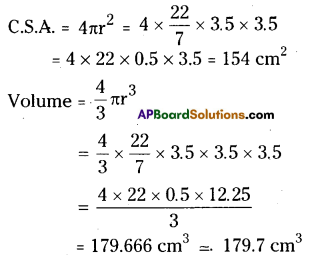Question 2.
The surface area of a sphere is 1018$$\frac{2}{7}$$ cm2 . What is its volume ?
Solution:
Surface area of sphere = 4πr2
= 1018$$\frac{2}{7}$$ cm2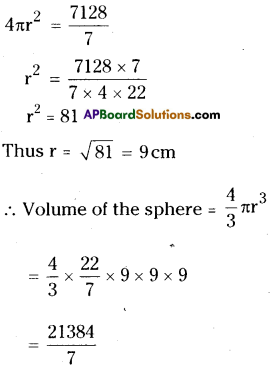= 3054.857cm3
≅ 3054.86cm3

Question 3.
The length of equator of the globe is 44 cm. Find its surface area.
Solution:
Length of the equator of the globe 2πr = 44 cm.
2 × $$\frac{22}{7}$$ × r = 44
∴ r = $$\frac{44 \times 7}{2 \times 22}$$ = 7cm
∴ surface area = 4πr2
= 4 × $$\frac{22}{7}$$ × 7 × 7
= 4 × 22 × 7
= 616cm2Question 4.
The diameter of a spherical ball is 21 cm. How much leather is required to prepare 5 such balls?
Solution:
Diameter of the spherical ball d’ = 21 cm
Thus, its radius r = $$\frac{d}{2}=\frac{21}{2}$$ = 10.5 cm
Surface area of one ball = 4πr2
= 4 × $$\frac{22}{7}$$ × 10.5 × 10.5
= 88 × 1.5 × 10.5 = 1386 cm2
∴ Leather required for 5 such balls
= 5 × 1386 = 6930 cm2

Question 5.
The ratio of radii of two spheres is 2 : 3. Find the ratio of their surface areas and volumes.
Solution:
Ratio of radii r1 : r2 = 2 : 3
Ratio of surface area
= 4πr12 : 4πr22
= 22: 32 = 4 : 9
Ratio of volumes
= 4/3 πr13 : 4/3 πr23
= 23 : 33 = 8 : 27

Question 6.
Find the total surface area of hemisphere of radius 10 cm. (Use π = 3.14)
Solution:
Radius of the hemisphere = 10 cm
Total surface area of the hemisphere = 3πr2
= 3 × 3.14 × 10 × 10
= 9.42 × 100
= 942 cm2Question 7.
The diameter of a spherical balloon increases from 14 cm. to 28 cm. as air is being pumped into it. Find the ratio of surface areas of the balloons in the
two cases.
Solution:
The diameter of the balloon, d = 14 cm
Thus, its radius, r = $$\frac{d}{2}=\frac{14}{2}$$ = 7 cm
∴ Surface area = 4πr2 = 4 × $$\frac{22}{7}$$ × 7 × 7
= 88 × 7 = 616cm2
When air is pumped, the diameter = 28 cm
thus its radius = $$\frac{d}{2}=\frac{28}{2}$$ = 14 cm
Its surface area = 4πr2
= 4 × $$\frac{22}{7}$$ × 14 × 14
= 88 × 28 = 2464 cm2
Ratio of areas = 616 : 2464
= 1 : 4

(OR)

Original radius = $$\frac{14}{2}$$ = 7 cm
Increased radius = $$\frac{28}{2}$$ = 14cm
Ratio of areas = r12 : r22
= 72 : 142
= 7 × 7 : 14 × 14
= 1:4Question 8.
A hemispherical bowl is made of brass, 0.25 cm thickness. The inner radius of the bowl is 5 cm. Find the ratio of outer surface area to inner surface area.
Solution:
Inner radius of the hemisphere ‘r’ = 5 cm
Outer radius of the hemisphere ‘R’
= (5 + 0.25) cm = 5.25 cm
Ratio of areas = 3πR2: 3πr2
= R2 : r2
= (5.25)2: 52
= 27.5625 : 25
= 1.1025:1
= 11025 : 10000
= 441 : 400

Question 9.
The diameter of a lead ball is 2.1 cm. The density of the lead used is 11.34 g/c3. What is the, weight of the ball ?
Solution:
The diameter of the ball = 2.1 cm
Thus, its radius, r = $$\frac{d}{2}=\frac{2.1}{2}$$ = 1.05 cm
Volume of the ball V’ = $$\frac{4}{3}$$πr3
= $$\frac{4}{3} \times \frac{22}{7}$$ x 1.053 = $$\frac{101.87}{21}$$
∴Weight of the ball = Volume × density
= 4.851 × 1.34
= 55.010Question 10.
A metallic cylinder of diameter 5 cm 1 and height 3 $$\frac{1}{3}$$ cm is melted and cast into a sphere. What is its diameter ?
Solution:
Diameter of the cylinder’d’ = 5 cm
Thus, its radius, r = $$\frac{d}{2}=\frac{5}{2}$$ = 2.5 cm
Height of the cylinder,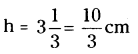Volume of the cylinder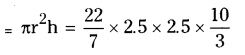Given that cylinder melted to form sphere
∴ Volume of the sphere = Volume of the cylinder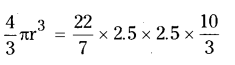(Where r is the radius of the sphere)
r3 = $$\frac{3}{4}$$ × 2.5 × 2.5 × $$\frac{10}{3}$$
r3 = 2.53
∴ r = 2.5 cm
Hence its diameter, d = 2r
= 2 × 2.5 = 5 cmQuestion 11.
How many litres of milk can a hemispherical bowl of diameter 10.5 cm hold ?
Solution:
Diameter of the hemispherical bowl = 10.5 cm
Thus its radius = $$\frac{d}{2}=\frac{10.5}{2}$$ = 5.25cm
Quantity of milk, the bowl can hold = Volume of the bowl = $$\frac{2}{3}$$πr3
= $$\frac{2}{3} \times \frac{22}{7}$$ × 5.25 × 5.25 × 5.25
= 303.1875 cm3
= $$\frac{303.1875}{1000}$$ lit = 0.303 lit.

Question 12.
A hemispherical bowl has diameter 9 cm. The liquid is poured into cylindrical bottles of diameter 3 cm and height 3 cm. If a full bowl of liquid is Riled in the bottles, find how many
bottles are required ?
Solution:
Diameter of the hemispherical bowl ‘d’ = 9 cm
Its radius, r = $$\frac{d}{2}=\frac{9}{2}$$ = 4.5cm
Volume of its liquid = Volume of the bowl = $$\frac{2}{3}$$ πr3
= $$\frac{2}{3} \times \frac{22}{7}$$ × 4.5 × 45 × 4.5
Diameter of the cylindrical bottle, d = 3 cm
Its radius, r = $$\frac{d}{2}$$
= $$\frac{3.0}{2}$$
= 1.5cm

Height of the bottle, h = 3 cm
Let the number of bottles required = n
Then total volumes of these n bottles = n πr2h
But this is equal to volume of the bowl
Hence n. $$\frac{22}{7}$$ × 1.5 × 1.5 × 3
= $$\frac{2}{3} \times \frac{22}{7}$$ × 4.5 × 4.5 × 4.5
∴ n = $$\frac{2}{3} \times \frac{20.25}{1.5}$$ = 9
∴ Number of bottles required = 9